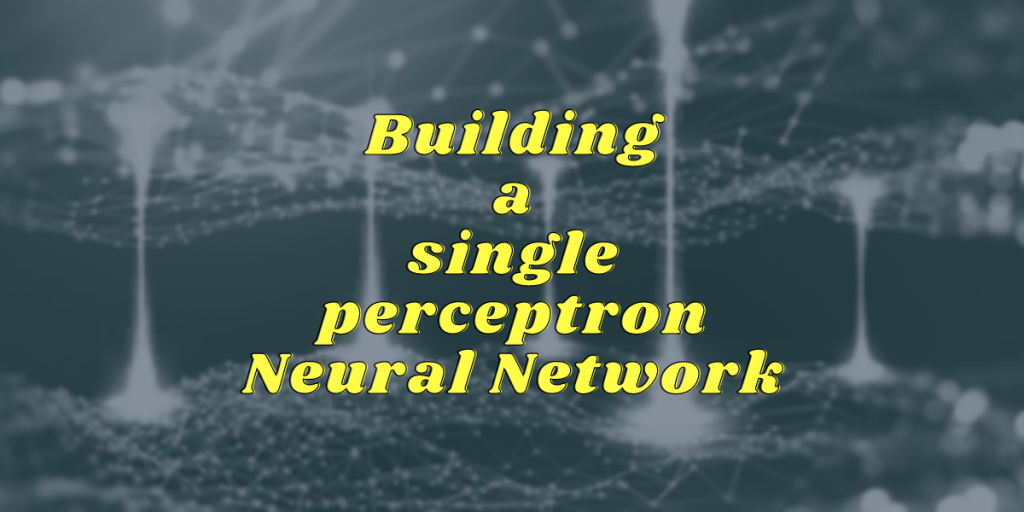# Building a Single Perceptron Neural NetworkWelcome. Today, we are going to cover how to build a basic single perceptron neural network.

I want to make this the first of a series of articles where we delve deep into everything – CNNs, transfer learning, etc. so be sure to bookmark the site and keep checking it. Also, this will include some math, so strap in.

## What is Perceptron?

It is the most fundamental unit of a neural network (NN). In a complex NN, all data pass through several of these, often at the same time and performing different roles. But we’ll get to that later.

For now, what is a perceptron?

In supervised learning, a perceptron is a form of a linear classifier. So, any dataset that can be divided by a single straight line can be classified using a perceptron, i.e., any dataset that looks like this:

The last one cannot be considered linearly separable, because it is not a single line that can approximately separate the database.

## Building A Single Perceptron Neural Network

Let’s move on to building our first single perceptron neural network today. For this, we’ll begin with creating the data.

### 1. Create our dataset

First, we need our data set, which in our case will a 2D array. Open up your code editors, Jupyter notebook, or Google Colab.

```import pandas as pd
import numpy as np
import random
```

Let’s make our data. I consider a 20*20 plane in this example to keep our results small and concise.

```#Dataset
df = pd.DataFrame()
df['x'] = [random.randint(1,20) for x in range(10)]
df['y'] = [random.randint(1,20) for x in range(10)]
```

Now, we need to label these. So we’ll filter out based on a line (I considered y=x). So every point below the line is y<x and every point above the line is mean y>x.

```label=[]
for i in range(df.shape):
if df.iloc[i,0] < df.iloc[i,1]:
label.append(1)
else:
label.append(-1)

df['label'] = label
df
```Dataset With Labels For Neural Nets Perceptron Model

### 2. Initialize weights for the values

Now we can initialize the weights. We can’t use zero value, so we’ll just go with a random uniform distribution for weights:

```#weights and bias
weights = [np.round(random.uniform(-0.99,0.99),2) for i in range(2)]
```

Then we multiply the weights with the input data points and sum:

```w = weights.copy()
X = [[df.iloc[i,0],df.iloc[i,1]] for i in range(df.shape)]
wx = [X[i]*w+X[i]*w for i in range(df.shape)]
```

Now, we have the sum of weights and inputs for each point.

So what the procedure is, is that we’ll plug in all these values one at a time into the activation function, and then based on output we’ll modify the weights.

### 3. Creating the Activation Function

Now we come to the activation function. The perceptron treats the sum and gives us a label, which we compare with the original label and determine if it is correct. If it is incorrect, the error is found and the weights are adjusted so that our solution *moves* in the direction of the original.

We’ll be using the signum function:

If wx <=0 , then output is 0. Else, the output is 1.

```for i in range(df.shape):
if wx[i]<=0:
pred = 0
else:
pred = 1
```

Remember, we only have two inputs: x and y. Not the whole dataframe. So, we will expand this activation function to take in one datapoint at a time, and then finding the error and then adjusting the error:

```for i in range(df.shape):
if wx[i]<=0:
pred = -1
else:
pred = 1
if pred != df['label'][i] :
err = df['label'][i] - pred
w = w + err
w = w + err
```

This works perfectly now. To clearly see the outputs, we’ll put in a bunch of print statements:

```for i in range(df.shape):
print('wx : ',wx[i])
if wx[i]<=0:
pred = -1
else:
pred = 1
print('label=',df['label'][i])
print('pred = ',pred)
if pred != df['label'][i] :
err = df['label'][i] - pred
print('err',err)
print('before', w,w)
w = w + err
w = w + err
print('after',w,w)
else:
print('w_i', w,w)
```

And now if we run this:Updating Weights Single Perceptron Neural Network

A simple print formatting statement gives us the final weights.Final Weights Single Perceptron Neural Network

### 4. Testing our model on another database

Similar to how we did the train-test-split, we’ll use a different database for our testing here.

```#Test Dataset
new_df = pd.DataFrame()
new_df['x'] = [random.randint(1,20) for x in range(100)]
new_df['y'] = [random.randint(1,20) for x in range(100)]
```

Then we generate the labels based on y=x line, and the sum of weights*inputs:

```label_text = []
for i in range(new_df.shape):
if new_df.iloc[i,0] < new_df.iloc[i,1]:
label_text.append(1)
else:
label_text.append(-1)
new_wX = w*new_df['x']+w*new_df['y']
```

Here is mine:

So, now the moment of truth, we apply our activation function, and then we can compare the given labels with the predicted labels:

```new_df['given label'] = label_text
pred_label_text = []

for i in range(new_df.shape):
if new_wX[i]>=0:
pred_label_text.append(-1)
else:
pred_label_text.append(1)

new_df['predicted labels'] = pred_label_text
```